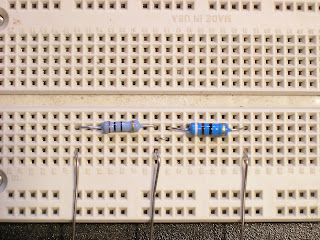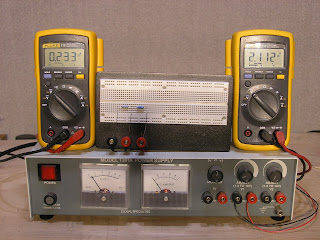## Friday, January 30, 2009

### What is a voltage divider? Pt 2

To help visualize the concept from part 1 of this post, here's a quick circuit mock up of the circuit diagrammed there. On the left is a 10 ohm resistor with the left hand meter's probes connected across it. Connected in series to this resistor is a 90 ohm one with the right hand meters leads connected across it.Below the meters are reading the actual values of their respective resistors (10.4 ohms to the left and 91.4 ohms to the right). This works out to pretty much the 10% and 90% of total resistance as shown in part 1.Now the power supply is connected to the circuit and turned on.The left hand resistor is seeing o.233 volts and the right hand one is seeing 2.112 volts. The sum of the voltage drops across the two resistors is 2.345 volts.

o.233 divided by 2.345 is .101 or about 10%
2.112
divided by 2.345 is .901 or about 90%The voltage is raised to about 4.5 volts on the power supply.

Now the left hand resistor is seeing 0.439 volts and the right hand one is seeing 3.99 volts. The sum of those voltage drops 4.429 volts.

0.439 divided by 4.429 is .099 or about 10%
3.99
divided by 4.429 is .903 or about 90%The voltage is raised again to about 10 volts on the power supply.

Now the left hand resistor is seeing 1.010 volts and the right hand one is seeing 9.05 volts. The sum of those voltage drops 10.06 volts.

1.01 divided by 10.06 is .1 or about 10%
9.05
divided by 10.06 is .899 or about 90%

So no matter how the voltage changes the ratio between the voltages across the resistors stays the same.

With an output connected across the 90 ohm resistor (as shown in part 1), any voltage input to the circuit will produce 90% of that voltage at the output. Now you can see how the voltage divider is effective for the constantly changing AC signal generated by your guitar as well as for a simple DC circuit.